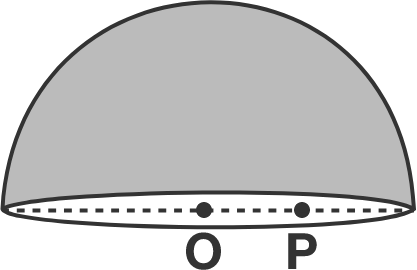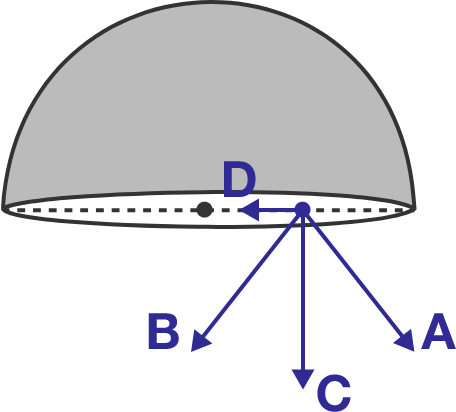# Electric BodyA thin, non-conducting hemispherical shell contains a positive charge $Q$ on it, which is uniformly distributed on the shell. Point $\text{O}$ is the center of the base circle, and point $\text{P}$ is on a diameter of the base circle some distance away from $\text{O}.$

Which of the vectors shown below represents the direction of the electric field at point $\text{P}?$×